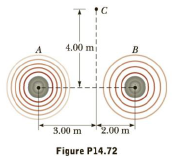Chapter 14, Problem 72AP

Chapter
Section
Textbook Problem

Two small loudspeakers emit sound waves of different frequencies equally in all directions. Speaker A has an output of 1.00 mW, and speaker B has an output of 1.50 mW. Determine the sound level (in decibels) at point C in Figure P14.72 assuming (a) only speaker A emits sound, (b) only speaker B emits sound, and (c) both speakers emit sound.(a)

To determine
The sound level in (decibels) at point C due to speaker A alone.

Explanation

Given Info: The distance of the sound waves at the point C from the centre axis of the two speakers is 4.00m and the distance of the speaker A to the middle of point C is 3.00m .

Formula to calculate the distance from speaker A using Pythagoras theorem is, rA=r12+rC2

• rA is the distance from speaker A to the point C,
• rC is the distance of the point C,
• r1 is the distance of the speaker A,

Substitute 3.00m for r1 and 4.00m for rC .

rA=(3.00m)2+(4.00m)2=5.00m

Thus, the distance from speaker A to the point C is 5.00 m.

Formula to calculate the sound intensity for the speaker A alone is,

IA=PAA2

• IA is the sound intensity for the speaker A,
• PA is the output power of the speaker A,
• A is the area,

Use 4πrA2 for A in the above relation.

IA=PA4πrA2

Substitute 1.00mW for PA and 5.00m for rA , we get IA .

IA=(1

(b)

To determine
The sound level in (decibels) at point C due to speaker B alone.

(c)

To determine
The sound level in (decibels) at point C due to both speakers emit sound.

Still sussing out bartleby?

Check out a sample textbook solution.

See a sample solution

The Solution to Your Study Problems

Bartleby provides explanations to thousands of textbook problems written by our experts, many with advanced degrees!

Get Started